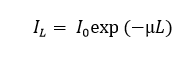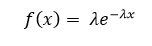## Saturday, April 26, 2014

### The Exponential Distribution

The exponential distribution holds a special significance for me. My PhD thesis was all about optical transients, the simplest mathematical models of which are exponential distributions. Currently, I work in x-ray science, which is heavily concerned with the depletion of an (x-ray) optical field as it traverses some distribution of matter (both in an object being imaged, and in the detector) - this time the exponential distribution is over space, rather than time, but the mathematics is the same.

Any kind of involvement with mathematical science quickly brings us into intimate contact with exponential functions, as these arise left, right, and centre, in the solutions of differential equations. The reason for this is related to the fact that the exponential is the only mathematical function that is its own derivative. This is closely related to a special property of the exponential distribution, known as memorylessness (what will happen next - its rate of change - is entirely governed by the current state). So let's take a quick look into how the exponential distribution comes about, and what its major characteristics are.

Imagine a stream of photons incident on some distribution of matter. It's no surprise to learn that some of those photons are going to be absorbed or scattered, so that they non-longer continue on their original path. The number that are scattered will depend on the thickness of the matter that they pass through, which is why, on a foggy day, things that are close to you can be easily seen, things not too far off can be somewhat made out, while objects a bit further away can't be seen at all. We'd like to know exactly what the dependence on distance is.

Lets denote as PL(U) the probability (dependent on length, L) that a photon will remain unabsorbed by its surrounding medium. For any infinitessimally thin strip of that medium (whose distance in is L), the probability to be absorbed at that location is the product PL(U) × P(A | U), where P(A | U) is the probability to be absorbed in that strip, given that it was not absorbed in any early strip. This follows from the product rule applied to the necessary conjunction, 'unabsorbed, before now' AND 'absorbed here', required for an absorption to occur at a particular place. The probability P(A | U) is independent of where the photon has been up to now - adding the U after the vertical bar ensures this. There is no physical reason for P(A | U) to depend on the photon's history, and this is the property of memorylessness I mentioned a moment ago. To put it another way, we are dealing with a Markov process, which can be a useful fact to remember.

Because P(A | U) is unchanging, we have invented a special symbol for it, μ, which we call the absorption coefficient. As each consecutive layer of the absorbing medium is traversed by the photon, the probability for the photon not to have been absorbed is reduced by the amount, PL(U) × μ (from the sum rule). Or, to put it another way, the rate of change of PL(U) with respect to the path length traversed is:(1)
We can rearrange this equation, then take the integral of each side:

(2)

The left-hand side is solved using item 5 in my table of intergals, while the right-hand side is given by item 3:(3)
As always, ln(.) represents the natural logarithm. Since this equation is true for all distances, we can form equations for distances, L and 0, and then subtract the 2 equations:(4)

From the laws of logs, this becomes(5)
or, taking the exponential of each side(6)
where we have finally identified the proportionality of P(U) and the intensity, I, of the optical field. This describes the exponential decay of the photon flux. This equation is called the Beer-Lambert Law. PL(U) is not a probability distribution over L, however, as the set of propositions, unabsorbed at L1, unabsorbed at L2, ... etc., are not exclusive. PL(A), though, is a distribution over a set of disjoint (non-overlapping) propositions (a photon can not be absorbed in more than one place), and as we found, is proportional to PL(U). As noted above, the constant of proportionality is μ, so the absorption probability density as a function of distance, L, is (setting the photon's initial existence probability, I0, to 1):(7)
It's easy enough to verify that this function is normalized (e.g. check Eq. 12, for L = ∞).

In general, if a parameter, x, is assigned an exponential distribution, with decay constant, λ, then the normalized PDF is(8)
To maintain unit consistency, the units of λ are the inverse of the units of x. If x is distance, in mm, then λ has units mm-1. If x is time, in seconds, then λ is a rate (or frequency) with units s-1.

Below, I've plotted an exponential decay (not normalized), following exp(-x/300), from x = 0 to 1000:

We can visualize the memorylessness of the thing, and appreciate how some of the exponential distribution's spooky symmetry comes about by starting at any point further along the x-axis and advancing along x by a distance of another 1000 units, and expanding the y-axis to fill the same amount of space on the screen. Below, I chose to start at x = 900, near the end of the previous plot. The curve looks identical to before. Note that the numbers on the x- and y-axes are different, but the functional form is the same. It is as if those first 900 units on the x-axis had never happened.

Any two-level, time-invariant decay process is exponential. The photon is a two-level system, it goes from unabsorbed to absorbed, then it's game over, and as long as its environment isn't changing, it exhibits the required temporal symmetry. A radioactive nucleus is a similar two level system - not decayed followed by decayed. Very many other physical systems follow the same pattern. The process is still exponential if there are several decay channels between the two levels of the system. More complex dynamics can be described by various combinations of exponential functions.

Beyond photons and atoms, many other phenomena are exponential. Even some human affairs, such as the time that a hospital bed remains occupied, follow this remarkable formula.

The mean of the exponential distribution is obtained in the usual way, by evaluating the definite integral from 0 to ∞ (the exponential distribution has no density below x = 0):
(9)

This is can be tackled easily using integration by parts, yielding

(10)

In another amazing display of symmetry, the standard deviation for the exponential distribution is the same as the mean:

(11)

Obtaining the cumulative distribution function for the exponential distribution is as easy as it ever gets. Where f(L) = μ × exp(-μL) was the probability for a photon to be absorbed at L (Eq. 7), recall that exp(-μL) was also the probability for the photon to be unabsorbed prior to reaching L. But the statement 'unabsorbed up to L' is complimentary to the statement, 'absorbed anywhere between 0 and L,' so the CDF is simply(12)

When an electron in an atom is given a jolt of extra energy, and promoted to a higher orbital, the time in which is stays in that high energy state, before relaxing down to its equilibrium state also follows the exponential distribution. The average lifetime of the excited state is 1/λ, which is termed the time constant, τ, and the evolution of an ensemble of N excited atoms is written
(13)

It is straightforward to see that τ is the expected time it takes for the number of excited atoms to fall to 1/e times the initial number.

Radioactive nuclei are more usually characterized by their half life, T1/2, rather than τ. The half life is the time it takes N(t) to reach half its initial value. It is the median of the exponential distribution, as can be seen directly from Eq. 12. It is found easily by setting t = T1/2 in Eq. 13: N(T1/2) / N(0) = exp(-T1/2/τ) = 1/2, and solving:
(14)

This particular formula highlights the general difference between the mean and the median: the mean is the centre of mass (depending on the sum of the products of mass times distance), while the median is the point at which the mass to the left equals the mass to the right (depending only on the sum of the masses).

Note: we mustn't fall into the trap of thinking that after 2 half lives, all the radioactive nuclei will have decayed. Remember, the process is memoryless - in 2 half lives, the population drops to one quarter, in 3 half lives it drops to one eighth, and so on.

Of further interest is that for any continuous parameter restricted to non-negative values, the exponential distribution has the property of maximum entropy.